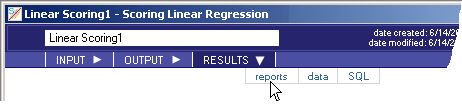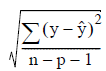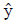# 5.4.5 - Linear Scoring - RESULTS - Reports - Teradata Warehouse Miner

## Teradata Warehouse Miner User Guide - Volume 3Analytic Functions

Product
Release Number
5.4.5
Published
February 2018
Language
English (United States)
Last Update
2018-05-04
dita:mapPath
yuy1504291362546.ditamap
dita:ditavalPath
ft:empty
1. On the Linear Scoring dialog box, click RESULTS.
2. Click reports (note that the RESULTS tab is disabled until after the analysis is completed).
Linear Scoring > Results > Reports• Resulting Scored Table — Name of the scored table - equivalent to Result Table Name.
• Number of Rows in Scored Table — Number of rows in the Resulting Scored Table.
• Evaluation
• Minimum Absolute Error
• Maximum Absolute Error
• Average Absolute Error

The term error in the evaluation of a linear regression model refers to the difference between the value of the dependent variable predicted by the model and the actual value in a training set of data (data where the value of the dependent variable is known). Considering the absolute value of the error (changing negative differences to positive differences) provides a measure of the magnitude of the error in the model, which is a more useful measure of the model’s accuracy. With this introduction, the terms minimum, maximum and average absolute error have the usual meanings when calculated over all the observations in the input or scored table.

• Standard Error of Estimate — The standard error of estimate is calculated as the square root of the average squared residual value over all the observations, i.e.,where y is the actual value of the dependent variable,is its predicted value, n is the number of observations, and p is the number of independent variables (substitute n-p in the denominator if there is no constant term).